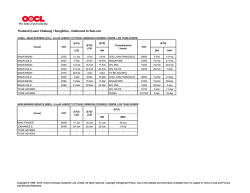# "Factorization of holomorphic eta quotients"

```Seminario congiunto
Dipartimento di Matematica – Centro Internazionale per la Ricerca Matematica
martedi' 3 febbraio ore 14:00
Aula Seminari – Dipartimento di Matematica
Factorization of
holomorphic eta quotients
ABSTRACT:
Soumya Bhattacharya
(CIRM-Trento) Borsista Post Doc del
CIRM per l'anno 2015
Unlike integer factorization, a reducible holomorphic
eta quotient may not factorize uniquely as a product of
irreducible holomorphic eta quotients.
We conjecture the occurrence of a certain type of
factor of a holomorphic eta quotient whenever it is
reducible and we give a short sketch of our proof of
this conjecture for all prime power levels. This also
implies that rescaling and Atkin-Lehner involutions of
irreducible holomorphic eta quotients of prime power
levels are irreducible. In particular, our conjecture
implies an irreducibility-checking algorithm for
holomorphic eta quotients.
We show that even without assuming this conjecture,
irreducibility of an eta quotient could be checked in
finite time.
We also show that there are finitely many simple
holomorphic eta quotients of a given level and provide
a bound on the weights of such eta quotients of a given
level.
Finally, we construct an infinite family of irreducible
holomorphic eta quotients of prime power levels.
Referente: Marco Andreatta
STAFF DIPARTIMENTO DI MATEMATICA
Università degli Studi Trento
Via Sommarive, 14- 38123 Povo (TN)
Tel +39 0461/281508-1625-1701 Fax+ 39 0461/281624
dept.math@unitn.it
```